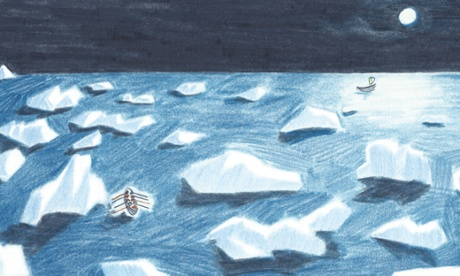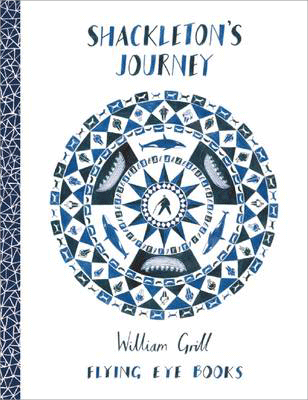,
Message sent from:

Welcome to Eagle Class! (Year 5/6)

Mrs Hotchin (class teacher)  and Miss Morley (teaching assistant)

Welcome to Spring term! Our topic is 'Frozen Kingdom'.We shall be learning all about the journey taken by Ernest Shackleton, and understand the dangers he faced by exploring and researching the climate of Antarctica.

### Our weekly maths overviews. Tasks will be set on MyMaths and other tasks are available on Mathletics.

Eagle class maths week beginning 10 2 20

Y6:

I can calculate %.

I can find the whole amount when I know a %.

I can calculate % increases and decreases.

Y5:

I can use long multiplication.

I can solve problems involving long multiplication.

I can divide 4 digits by 1 digit.

I can interpret remainders.

Eagle class maths week beginning 3.2.20

Y6: I can find fraction, decimal and % equivalences; I can order fractions, decimals and %; I can find % of amounts; I can find the whole when I know a %.

Y5: I can find fraction, decimal and % equivalences; I can use long multiplication.

Eagle class maths week beginning 27th January 2020

Y6: I can understand %; I can find fraction, decimal and % equivalences through conversion.

Y5: I can round decimals to the nearest tenth; I can understand the term 'percent'; I can find simple fraction and % equivalences.

Eagle class maths week beginning 20.1.20

Y5: (the children will be undertaking some assessments this week); I can understand thousandths; I can order decimals.

Y6: I can divide decimals; I can solve problems involving division; I can show decimals as fractions and vice versa.

Eagle class maths week beginning 13/1/20

Y5 - I can read and write decimals up to 3 decimal places.

I can understand the relationship between fractions and decimals.

I can solve problems involving decimals.

Y6- I can read and write decimals up to 3 decimal places.

I can multiply and divide decimals by 10, 100 and 1000.

I can multiply decimals by integers.

Eagle class maths week beginning 7.1.20

Y5- I can find fractions of amounts; I can use fractions as operators.

Y6- I can find fractions of amounts; I can find the whole when I know a fraction.

Eagle class maths week beginning 9.12.19

Y6- I can divide fractions by integers.

Y5- I can subtract mixed numbers; I can multiply a fraction by an integer.

### English

This half term we will begin by studying a range of different poetry with the theme of winter. We shall then be reading the book Shackleton's Journey, alongside 'Ice Trap' to gather information about Ernest Shackleton's voyage. We will be writing ship logs and newspaper articles.  We will also be studying a short story called ' A Night at the Frost Fair' and writing flashback stories.### Theme 'Frozen Kingdom'

In Geography we studying biomes, and the physical and human features of the polar biomes.

In art we shall be sketching snowflakes, and developing a design to create a batik pattern.

In DT we will be creating a recipe for a healthy snack for an explorer.

### In other subjects:

Science: Y6- light; Y5- materials

RE: Creation

Jigsaw: Unit : Goals

PE: gymnastics and invasion games